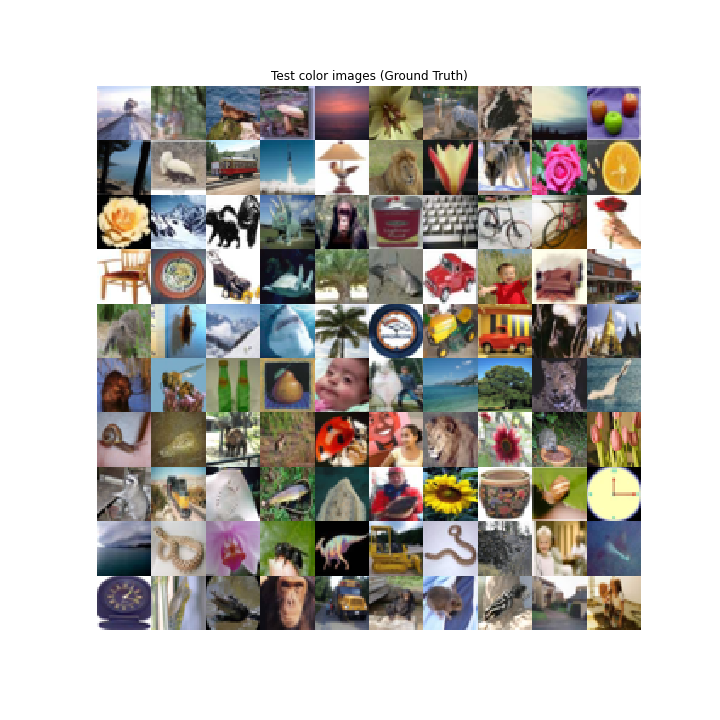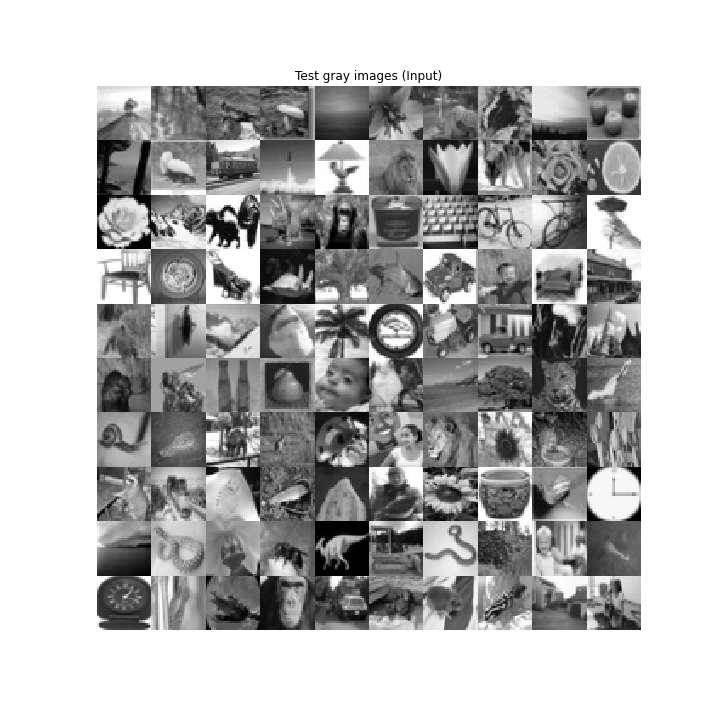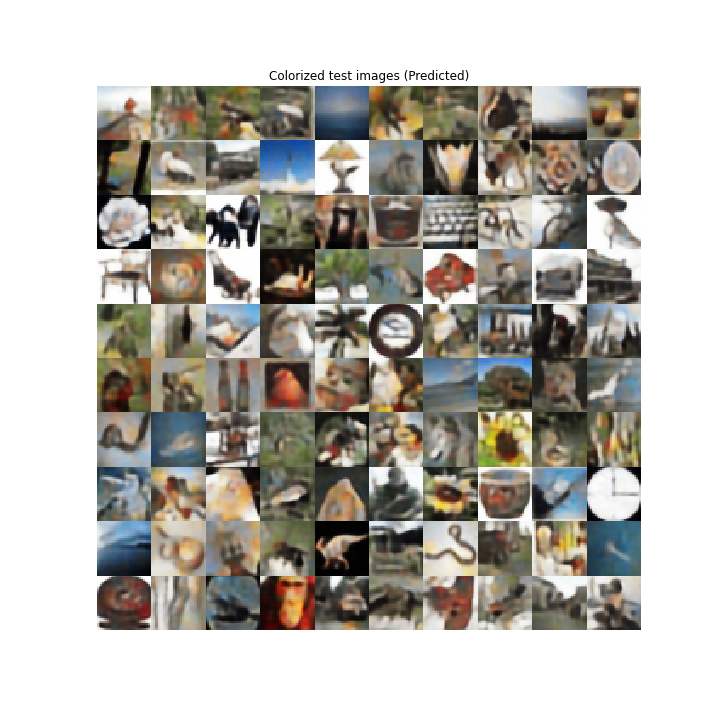# Colorization Autoencoders using Keras

This article gives a practical use-case of Autoencoders, that is, colorization of gray-scale images. We will use Keras to code the autoencoder.

As we all know, that an AutoEncoder has two main operators:

Encoder This transforms the input into low-dimensional latent vector.As it reduces dimension, so it is forced to learn the most important features of the input.
Decoder: This tries to reconstruct the input as much as possible from the latent vector.

During the design of Autoencoder, it is very essential to correctly choose a latent dimension. As if it is
more than the input dimension, Autoencoder tends to memorize the input. We will implement the Encoder part using CNNs and will use Conv2DTranspose for the decoder section of the autoencoder.

To keep things simple, we are going to use CIFAR100 dataset, which is readily available in Keras datasets
The dataset contains 50k colour images of shape 32 * 32 * 3 for training, and 10k colour images of the same shape for testing purpose.
Code: Import all the libraries

 `import` `numpy as np ` `import` `matplotlib.pyplot as plt ` `import` `os ` ` `  `from` `keras.layers ``import` `Dense, ``Input``, Conv2D, Conv2DTranspose, Flatten, Reshape ` `from` `keras.models ``import` `Model ` `from` `keras.callbacks ``import` `ReduceLROnPlateau, ModelCheckpoint ` `from` `keras.datasets ``import` `cifar100 ` `from` `keras ``import` `backend as K `

As the dataset contain only coloured images, so for the purpose of our task we need to change it to grey-scale. We hence define a function for that.
Code: Function to convert RGB images to Grayscale

 `def` `rgb_2_gray(image): ` `    ``return` `np.dot(image[..., :``3``], [``0.299``, ``0.587``, ``0.114``]) `

 `(x_train, _), (x_test, _) ``=` `cifar100.load_data() `

For the model to learn efficiently, it is better to convert the images into float. We also need to normalize the values so that they lie between 0 and 1. This is done so that during back-propagation, the gradients don’t go out of control.

Code: Normalize the data

 `x_train ``=` `x_train.astype(``'float32'``) ``/` `255.` `x_test ``=` `x_test.astype(``'float32'``) ``/` `255.` ` `  `x_train_gray ``=` `x_train_gray.astype(``'float32'``) ``/` `255.` `x_test_gray ``=` `x_test_gray.astype(``'float32'``) ``/` `255.`

Performance of Deep Learning models very much rely on the set of hyper-parameters (including no. of layer, no. of filters in each layer, batch size etc.). So a good choice of the hyper-parameters is an essential skill. For the best results, we need to try and experiment with a different set of them. Here, we are using these sets of hyper-parameters,
Code: Hyper-parameters

 `input_shape ``=` `(rows, cols, ``1``) ` `batch_size ``=` `32` `kernel_size ``=` `3` `latent_dim ``=` `256` `layer_filters ``=` `[``64``, ``128``, ``256``] `

For the task of colourizing, the input is a grey-scale image. Gray-scale image has only 1 channel as compared to colour images which have 3 namely Red, Green, Blue. We use Input from Keras library to take an input of the shape of (rows, cols, 1).
The Encoder is a stack of 3 Convolutional Layers with an increasing number of filters, followed by a Dense layer with 256 units for generating latent vectors.

Code: Encoder

 `inputs ``=` `Input``(shape ``=` `input_shape) ` `x ``=` `inputs ` `for` `filters ``in` `layer_filters: ` `  ``x ``=` `Conv2D(filters ``=` `filters, ` `           ``kernel_size ``=` `kernel_size, ` `           ``strides ``=` `2``, ` `           ``activation ``=``'relu'``, ` `           ``padding ``=``'same'``)(x) ` ` `  `shape ``=` `K.int_shape(x) ` `x ``=` `Flatten()(x) ` `latent ``=` `Dense(latent_dim, name ``=``'latent_vector'``)(x) ` `encoder ``=` `Model(inputs, latent, name ``=``'encoder'``) `

The decoder section of the Autoencoder tries to decompress the latent vector in order to the input. In our case, the input to the Decoder is a layer of shape (None, 256). It follows a stack of three DeConvolutional layers with decreasing filter numbers in each layer. We make sure that the last layer, in this case, should be of shape (None, 32, 32, 3). The number of channels should be 3 so as to compare the reconstruction with the ground truth of the images during the back-propagation.
It is not mandatory, that the Encoder and Decoder should be a mirror image of the two.

Code: Decoder

 `latent_inputs ``=` `Input``(shape ``=``(latent_dim, ), name ``=``'decoder_input'``) ` `x ``=` `Dense(shape[``1``]``*``shape[``2``]``*``shape[``3``])(latent_inputs) ` `x ``=` `Reshape((shape[``1``], shape[``2``], shape[``3``]))(x) ` `# stack of Conv2DTranspose(256)-Conv2DTranspose(128)- ` `# Conv2DTranspose(64) ` `for` `filters ``in` `layer_filters[::``-``1``]: ` `  ``x ``=` `Conv2DTranspose(filters ``=` `filters, ` `                      ``kernel_size ``=` `kernel_size, ` `                      ``strides ``=` `2``, ` `                      ``activation ``=``'relu'``, ` `                      ``padding ``=``'same'``)(x) ` `outputs ``=` `Conv2DTranspose(filters ``=` `channels, ` `                          ``kernel_size ``=` `kernel_size, ` `                          ``activation ``=``'sigmoid'``, ` `                          ``padding ``=``'same'``, ` `                          ``name ``=``'decoder_output'``)(x) ` `decoder ``=` `Model(latent_inputs, outputs, name ``=``'decoder'``) `

Finally, we define the model, named autoencoder which takes an input and then passes it to the encoder followed by passing it through the decoder.

 `autoencoder ``=` `Model(inputs, decoder(encoder(inputs)), ` `                    ``name ``=``'autoencoder'``) `

We now train the autoencoder model by slicing the entire data into batches of batch size = batch_size, for 30 epochs. The important point to note here is that, if we check out the of fit function, we find that, the input to the model is the dataset of grayscale images and the corresponding colour image is serving as the label. A similar thing happens for the validation set as well.
Generally, for the classification task, we feed the images to the model as inputs, and their respective classes are given as labels. and during training, we compare the performance of the model by measuring how well it classifies the images into their respective classes given as labels. But, for this task, we provide the colour images as the labels as we want the model to provide the RGB images as outputs when w provide a grey-scale image to it.
We have also used callbacks to reduce the learning rate if the validation loss is not decreasing much.

 `autoencoder.fit(x_train_gray, ` `                ``x_train, ` `                ``validation_data ``=``(x_test_gray, x_test), ` `                ``epochs ``=` `30``, ` `                ``batch_size ``=` `batch_size, ` `                ``callbacks ``=` `callbacks) `

Code: Results and analysisGround truth of the first 100 test imagesGrayscale input to the autoencoderColourized output from the Autoencoder

The autoencoder has an acceptable performance in the colourization job. It predicted correctly that the sky is blue, chimps have varying shades of brown, leaves are green etc. But also, it does some wrong predictions as well, like Sunflower has some shades of grey in it, orange has no colour predicted, the mushroom is dark and not reddish etc.

My Personal Notes arrow_drop_upCheck out this Author's contributed articles.

If you like GeeksforGeeks and would like to contribute, you can also write an article using contribute.geeksforgeeks.org or mail your article to contribute@geeksforgeeks.org. See your article appearing on the GeeksforGeeks main page and help other Geeks.

Please Improve this article if you find anything incorrect by clicking on the "Improve Article" button below.

Article Tags :
Practice Tags :

Be the First to upvote.

Please write to us at contribute@geeksforgeeks.org to report any issue with the above content.